﻿ 如何使用Python脚本语言的绘图库Matplotlib

如何使用Python脚本语言的绘图库Matplotlib

2019/7/11 1:15:16 人评论 次浏览 分类：PLC应用  文章地址：http://yunrun.com.cn/tech/2610.html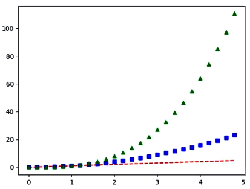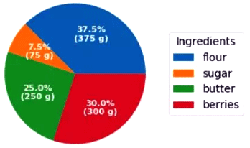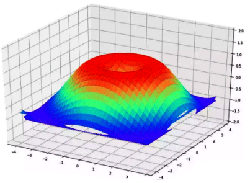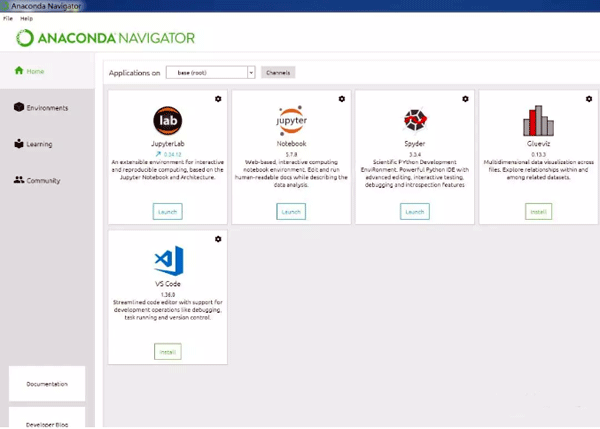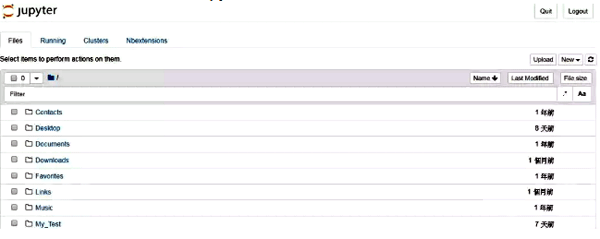import matplotlib.pyplot as plt #导入pyplot
import numpy as np #导入numpy
# np.linspace为numpy的等差数列函数，形成一个0到100之间，共50个元素的函数
x=np.linspace(0,100,50)
y=2*x+1 #赋值运算
#最重要的一步，plot用于绘制线条或标记的轴，可以指定线性，颜色等
plt.plot(x,y)  #默认直接绘制
plt.show()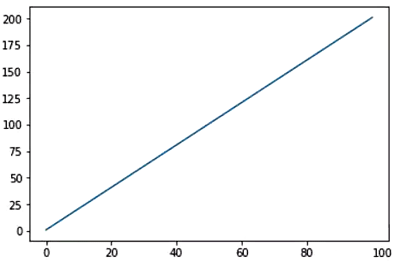import matplotlib.pyplot as plt
import numpy as np
x=np.linspace(0,100,20)
#print (x)
y=2*x+1
#与案例1中不同的是增加很多参数，如color(颜色), linewidth(线宽), linestyle(线性), marker(标记)
plt.plot(x,y,color='red',linewidth=1.0,linestyle='-',marker='o')
plt.show()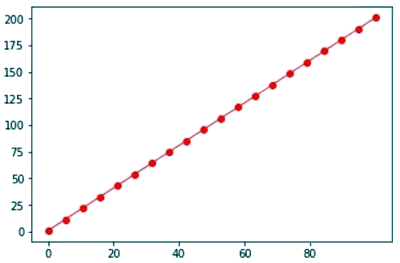import matplotlib.pyplot as plt
labels='Frogs', 'Hogs', 'Dogs', 'Logs' #定义标签
sizes=[15, 30, 45, 10] #定义饼图的大小
explode=(0, 0.1, 0, 0)  #定义切片
fig1, ax1 = plt.subplots() #导入饼图
#定义饼图的样式，此示例将 startangle设置为90 ，以便将所有对象逆时针旋转90度，定义了数
#据显示格式以小数显示，带阴影
ax1.pie(sizes, explode=explode, labels=labels, autopct='%1.1f%%',
ax1.axis('equal')  # 等纵横比可确保将饼图绘制为圆。.
plt.show()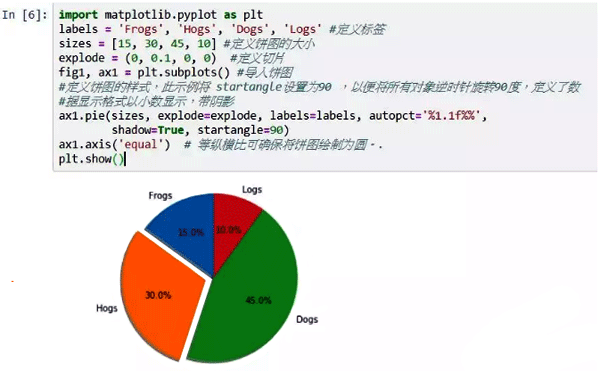import matplotlib.pyplot as plt
import numpy as np
from mpl_toolkits.mplot3d import Axes3D #导入3D图库
fig = plt.figure(figsize=(12, 8)) #指定figure的大小
ax = Axes3D(fig)
# 生成X，Y
X = np.arange(-4, 4, 0.25)
Y = np.arange(-4, 4, 0.25)
X,Y = np.meshgrid(X, Y)
R = np.sqrt(X**2 + Y**2)
# height value
Z = np.sin(R)
# 绘图
# rstride（row）和cstride(column)表示的是行列的跨度
ax.plot_surface(X, Y, Z,
rstride=1,  # 行的跨度
cstride=1,  # 列的跨度
cmap=plt.get_cmap('rainbow')  # 颜色映射样式设置
)
# offset 表示距离zdir的轴距离
ax.contourf(X, Y, Z, zdir='z', offest=-2, cmap='rainbow')
ax.set_zlim(-2, 2)
plt.show()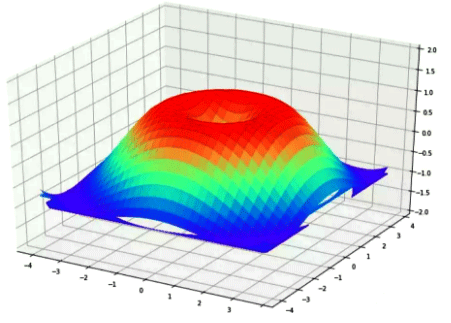与在线工程师QQ交流 到论坛与网友交流 向昌晖仪表网投稿 免费获得2000元电子书

客户姓名：